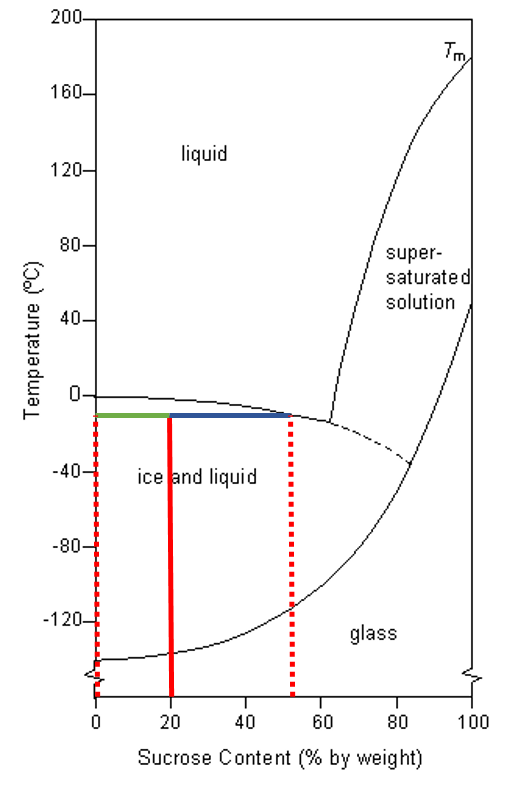# Phase Diagrams Explained – Demystifying Ice Cream

When you’re eating your ice cream, your caramel or a nice piece of fudge you might not realize that you’re actually consuming a scientifically highly complex product! The interactions between concentrations of sugar and water combined with temperature make for a great physical phenomenon. These are actually products which are great when represented in a so-called phase diagrams. These diagrams tell a lot about such a product.

I’ll give it a try to explain these phase diagrams, because they’re fascinating.

### What is a phase diagram

Let us start at the beginning, the definition of a phase diagram. In a phase diagram the phases of a material (or a mix of materials) is shown at different temperatures (and possibly pressures). Thus, in a phase diagram for water you would be able to see at which temperature it transitions from ice to water and from water to gas.

In this post we are not necessarily interested in a phase diagram of one material (e.g. water) but in a mixture of two components: for example sugar and water or salt and water.

The diagram below shows such a diagram. Here’s how to read them:

• On the horizontal axis you can find the concentration of salt (NaCl). The far right represents 100% salt, the far left represents 100% water.
• The vertical axis represents the temperature.
• The four different coloured areas represent the phases which are present at a specific temperature and concentration.

### Phase diagram of salt

Let us walk through two examples:

1. When the concentration of salt is 5% and the temperature is -10°C we end up in the red area: ice + salt water. This means that there is both salt water and ice in this mixture. Read more about this phenomenon in the post on ‘freezing point depression’. This red area explains why salt is spread on the roads in water.
2. In a salt solution with 50% salt at 5°C we end up in the grey area: NaCl crystals + salt water. This means that there are salt crystals inside a solution of salty water. This is what happens when you exceed the maximum concentration of salt in a solution. The salt doesn’t ‘fit’ anymore and crystallizes out.

### Phase diagram of sucrose

Since I have been discussing ice cream in a previous post in which sugar is commonly used to lower the freezing point, let’s also discuss the phase diagram of sucrose & water. The phase diagram shown below comes from the University of Cambridge website. For those familiar with the terms, it is a so called practical phase diagram.

Since we are talking ice cream here, we’ll only discuss one region. Take a sugar solution with 20% sucrose at -18°C, we end up in the ‘ice and liquid’ region. This means that there are both pure ice crystals as well as a solution of sugar. This is what makes our ice creams nice to eat, not to many ice crystals and sufficient liquid sugar solution which is ‘soft’.

### Calculating concentrations

There is one more important thing you can derive from a phase diagram: concentrations of the different phases. This is slightly more complex material so we will explain it using an example, use the graph below.

First, take a 100% water solution at -10°C (go up the far left red dotted line). Since there is only water, this phase will only exist of ice. Now take a 55% at the same temperature. You end up at the intersection between phase. This means that at this point there is only liquid (= sugar solution). Now let us take a point in the middle of these two.

Take a sugar solution with 20%  sugar at about -10°C. In the graph this is where the continuous red line ends. From this point two horizontal lines can be drawn to the closest boundaries. These lines represent the ratio of the liquid and ice phase. The green line indicates the liquid portion, the blue line is the ice fraction. If you ever forget which of the two lines is which, use the two examples I mentioned above.

The calculation here goes as follows:

• Fraction ice: length blue line / total length = (55-20)/55 = 0,64
• Fraction liquid: length green line / total length = (20-0)/55 = 0,36

Let’s check this. We said that the blue line is ice and the green line the liquid, so we should have more ice than liquid. This is exactly what we find in the calculation.Phase diagram of sucrose in water with the lines drawn out for the concentration calculation. Source

There is some complex mathematics underlying these calculations, but I decided to walk through that pretty quickly since it is not essential to understand the principles.

### Making a phase diagram

Unfortunately making a phase diagram isn’t very easy, especially when it comes to highly complex solutions. A solution of sugar and salt in water for instance is already a lot more complicated to visualize in such a diagram. If even more components are present, for example proteins, it becomes even more complex since they all interact.

Calculations of diagrams are often complex and we will not dive deeper into these details. However, once you know when material is in which phase you will be able to draw a phase diagram.

These phase diagrams come into play in a lot of different products and scenarios. When making sugar candy these diagrams are essential to understand and they help explain ice cream. Unfortunately, the more complex the mixture the harder it is to make exact calculations. This is why it is often hard to apply these diagrams one on one.

### References

University of Cambridge, water-sucrose systems, link

Harvard university, Science and cooking lecture series, link ; original link to phase diagram no longer active